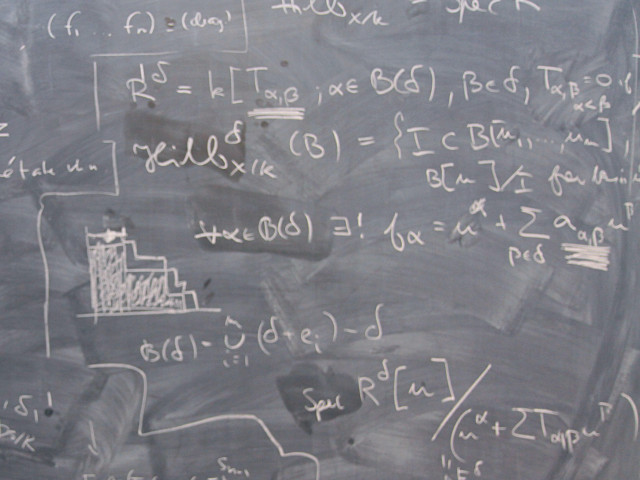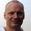### Choose semester and course offering

Choose semester and course offering to see information from the correct course syllabus and course offering.

## Content and learning outcomes

### Course contents

• Real and complex numbers.
• Functions and graphs.
• Trigonometric, exponential and logarithmic functions.
• Limits.
• Derivative, derivation rules and applications.
• Integral, rules of integration and applications.
• Equation solving.
• Extreme values
• Number sequences and series, convergence and divergence.
• Maclaurin and Taylor expansion.
• Differential equations.
• Iterative methods and numerical calculations.

### Intended learning outcomes

On completion of the course, the student should be able to:

1. Use, explain and apply the most important fundamental concepts and the problem solving methods from differential and integral calculus in a variable in particular:

• account for the basic properties of the elementary functions such as for example power laws, logarithm laws and trigonometric formulae, as well as use these in problem-solving and calculations
• calculate derivatives by means of e.g. the product rule, the quotient rule and the chain rule
• use the derivative to examine the properties of a function e.g. decide properties of growing and decreasing, draw the curve, decide tangent, prove inequalities and find extreme values
• use Taylor's formula to approximate functions with polynomial to given precision
• account for the definition and applications of the Riemann integral as well as approximate integrals with Riemann sums
• calculate integrals by means of primitive function, partial integration, substitution of variables and partial fraction decomposition
• account for the fundamental theorem of calculus about the relationship between derivative and integral of the analysis as well as use this in problem-solving and calculations
• solve a few types of linear ordinary differential equations with constant coefficients and account for how these arise in applications
• calculate limits and use these to study the behaviour of functions locally or asymptotically
• decide about a given function if it is invertible and if possible calculate the inverse
• decide if certain series are convergent or divergent and if possible calculate them.

2. Be familiar with the methodology for approximate or numerical solutions of equations and integrals and its application with mathematical software.

3. Set up simple mathematical models for applied processes that can be described by means of functions of one variable as well as discuss such models' relevance, reasonableness and precision.

4. Read and profit by mathematical text about functions of a variable and their applications as well as communicate mathematical arguments and calculations in this field orally and in writing in such a way that they are easy to follow.

### Course Disposition

No information inserted

## Literature and preparations

### Specific prerequisites

No information inserted

### Recommended prerequisites

High school mathematics, course "Matematik 4"

### Equipment

No information inserted

### Literature

No information inserted

## Examination and completion

If the course is discontinued, students may request to be examined during the following two academic years.

A, B, C, D, E, FX, F

### Examination

• TENB - Written Final Exam, 7,5 hp, betygsskala: A, B, C, D, E, FX, F

Based on recommendation from KTH’s coordinator for disabilities, the examiner will decide how to adapt an examination for students with documented disability.

The examiner may apply another examination format when re-examining individual students.

### Opportunity to complete the requirements via supplementary examination

No information inserted

### Opportunity to raise an approved grade via renewed examination

No information inserted

### ExaminerMattias Hammar

### Ethical approach

• All members of a group are responsible for the group's work.
• In any assessment, every student shall honestly disclose any help received and sources used.
• In an oral assessment, every student shall be able to present and answer questions about the entire assignment and solution.

## Further information

### Course web

Further information about the course can be found on the Course web at the link below. Information on the Course web will later be moved to this site.

Course web IX1304

### Offered by

EECS/Electrical Engineering

### Main field of study

Mathematics, Technology

First cycle